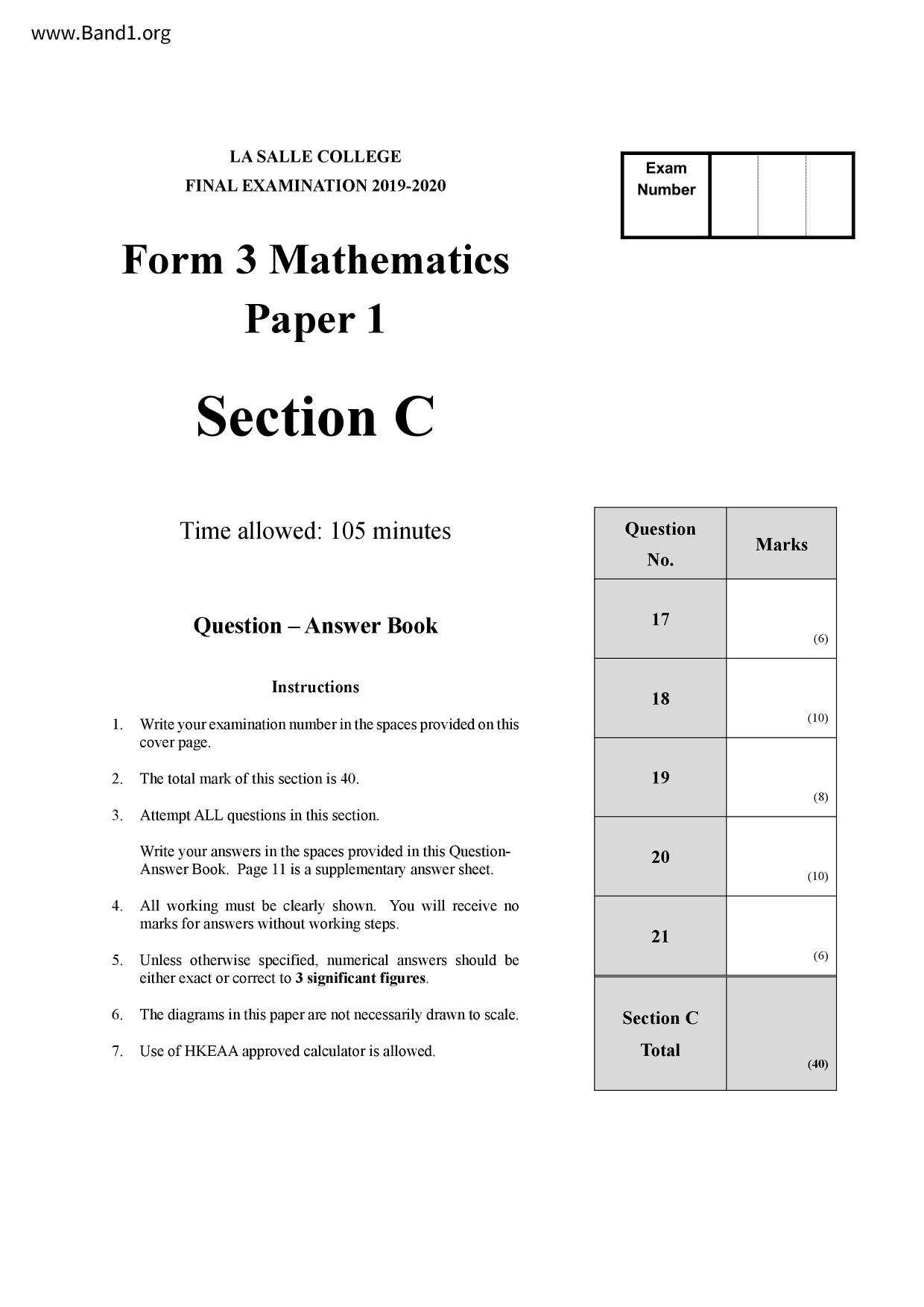# 中三 數學試卷 (F3 Maths Past Paper)

6631

pdf

12

F3 maths _F_IC_19_20

▼ 圖片只作預覽, 如欲下載整份卷, 請按「免費成為會員」 ▼▲ 圖片只作預覽, 如欲下載整份卷, 請按「免費成為會員」 ▲

## 中三數學試卷 PDF 下載

Form 3 Mathematics
LA SALLE COLLEGE
FINAL EXAMINATION 2019-2020
Time allowed: 105 minutes
Instructions
Write your examination number in the spaces provided on this
cover page.
3. Attempt ALL questions in this section.
The total mark of this section is 40.
All working must be clearly shown. You will receive no
marks for answers without working steps.
Unless otherwise specified, numerical answers should be
either exact or correct to 3 significant figures.
The diagrams in this paper are not necessarily drawn to scale.
7. Use of HKEAA approved calculator is allowed.
A hunter is stationing at the location A, targetting at a horse running from the location B
to the location C in a straight line as shown in the figure. It is known that B is due south
of A and C is S64°W from A. The distances of AB and AC are both 100 m.
What is the compass bearing of B from C?
The hunter is about to discharge the arrow from the bow such that the horse is hit
at the shortest distance from the hunter. The speeds of the horse and the arrow are
12 m/s and 80 m/s respectively.
(i) What is the shortest distance between the horse and the hunter?
Find the time the hunter has to wait for discharging the arrow when the horse
starts moving from B.
- End of Paper -
Section C (40 marks)
17. The frequency distribution table and the cumulative frequency distribution table below
show the distribution of the heights of the students in a class.
(a) Complete the tables below.
Height (cm)
Height less
Find an estimate of the mean of the distribution.
If a student is randomly selected, find the probability that the height of the selected
student is less than 170.5 but not less than 155.5.
18. In the figure, the straight line L₁: 3x - 4y - 24 = 0 cuts the x-axis at A and y-axis at B.
The straight line L2 is the perpendicular bisector of AB which cuts the x-axis at C and y-
axis at D. M is the intersecton of L₁ and L2.
Find the coordinates of A and B.
Find the area of AAMC.
L1:3x-4y-24=0
Find the equation of L2 in the form of y = mx + c.
In the figure, M and N are the mid-points of AD and BC respectively with AB // MN.
BD intersects AC and MN at P and Q respectively. AC intersects MN at R.
Prove that AB // DC.
Prove that A ABP ~ ACDP.
If PR = 6, BP =10, DP = 18, find AP.
A closed vessel in form of a right pyramid contains liquid to a depth of 4.5 cm as
shown in the figure. The bases of the frustum formed by the liquid are squares with
area 16 cm² and 256 cm² respectively. It is given that VX = x cm and PQ = y cm.
Find the values of x and y.
Show that h
Find the total surface area of the frustum.
The vessel is turned upside down such that the liquid forms a pyramid with
square base r cm and a depth of h cm.
(ii) Hence, find the value of r.